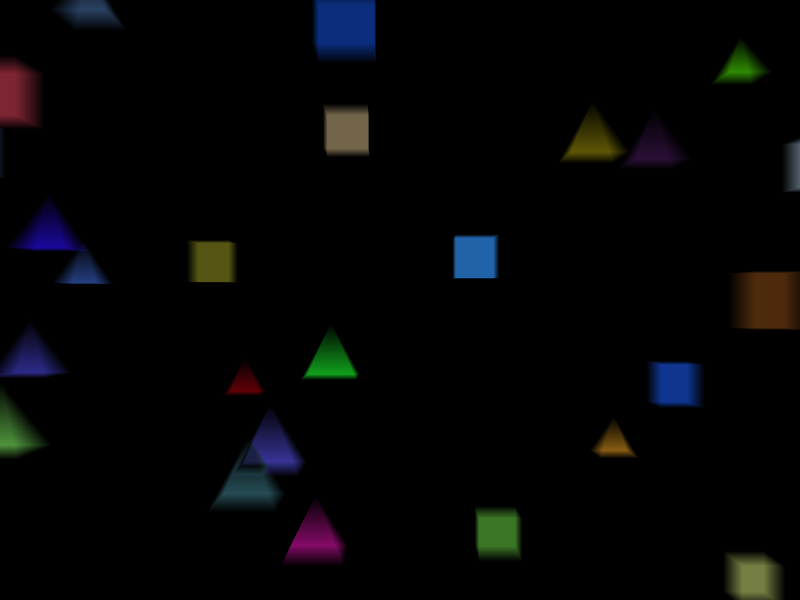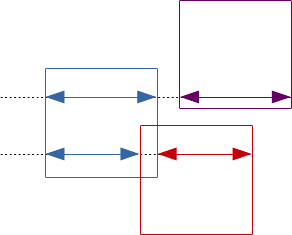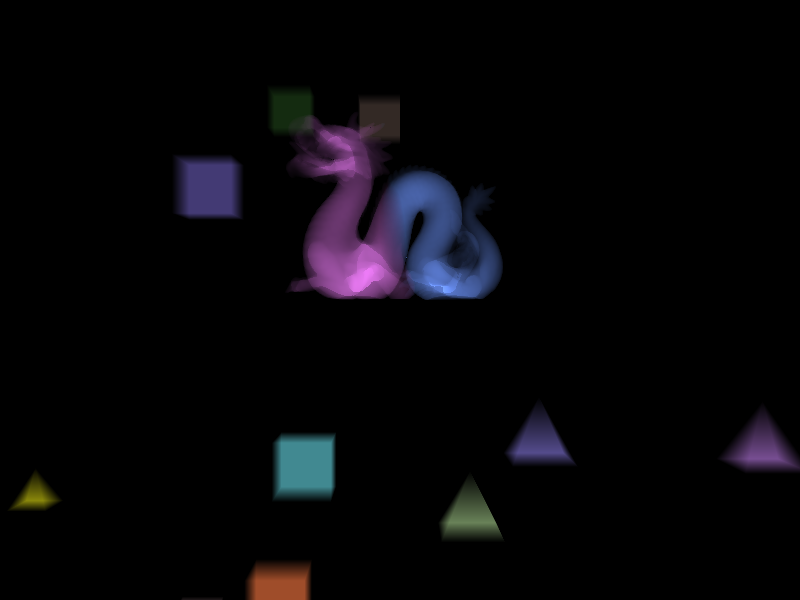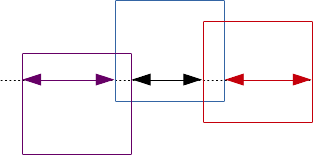# Depth Peeling Pseudo-Volumetric Rendering

### Matthew Wellings 25-Sept-2016

In my last blog post I described how Vulkan allows for practically efficient depth peeled order independent transparency. An interesting side effect of using this method is that we keep information that can be used for view space object depth calculations.
This allows us to draw transparent objects as if they are made of more than just thin layers such as the outer skin. Instead we can render them as if they are made of a solid translucent material but are in fact defined as a traditional triangle mesh. This method will not give an illusion of refraction or reflection. For that you may prefer a ray-tracing based method. It can however make objects appear as if they are made of aerogel or give the impression that you are looking at a sci-fi style hologram in your game.

### A Simple Method

In its simplest form we could draw every other layer and use a function of the difference of the current depth value and the previous value which are both still stored in depth buffers.
The depth values accessible in the fragment shader (both passed from the vertex shader and loaded from the depth buffer) are an inverse function of the view space depth. Using these values will cause objects that are further from the camera appear to be thinner than closer objects even though they are identical. In order to resolve this we must be able to restore the view-space depth from the clip-space depth equation. This can be derived using the projection matrix and must take in to account the Vulkan correction matrix.
The equation for view space depth from framebuffer space depth is: $\frac{nf}{f-n}/\left(z-\frac{f+n}{2\left(f-n\right)}+0.5\right)$ (view my derivation). If we take $a=\frac{nf}{f-n}$ & $b=\frac{f+n}{2\left(f-n\right)}+0.5$ then we have ViewDepth=a/(FBSDepth-b) with a & b both constants that can be computed outside of the shader.

This shader reads the two depth values, finds the view-space difference and uses a factor of this as the alpha:

``````#version 400

layout (input_attachment_index=0, set=2, binding=0) uniform subpassInput subpassInColour;
layout (input_attachment_index=1, set=3, binding=0) uniform subpassInput subpassInDepth1;
layout (input_attachment_index=2, set=4, binding=0) uniform subpassInput subpassInDepth2;
layout (location = 0) out vec4 outColor;

float viewSpaceDepth(float FBSDepth)
{
//((n*f)/(f-n))/(FBSDepth-((f+n)/(2*(f-n)))+0.5))
return 26.24/(FBSDepth-1.64);
}

void main() {
float depthdiff=viewSpaceDepth(lastDepth)-viewSpaceDepth(thisDepth);
float alpha = depthdiff/3.0;
outColor = vec4(color.r*alpha, color.g*alpha, color.b*alpha, alpha);
}``````

The folowing is a result of this simple method:There is however a notable problem with using this simple method. When two meshes overlap we can have undesirable results. It may be hard to define what the correct result is as the situation of two translucent materials occupying the same space is somewhat unphysical. It is important however that we can place two adjacent geometries together and not have catastrophic results from depth-fighting.

### Resolving overlapping geometry

In order that we handle overlap correctly we can use the information provided to the fragment shader as to whether we are rendering a front or back face. We can then create a stack which records the level of overlap and mixes colours appropriately for the desired scenario. For this demo I will consider the simple case of resolving close contact geometry which may overlap due to depth fighting.

In this case we only need to know which way the current surface is facing and which way the previous surface was facing. By storing the previous peeled colour buffer in the same way as we do with the depth buffer (as a pair of ping-ponged images) we can choose either the current or previous colour based on the current and previous face directions. The cases shown in the diagram below can be covered by choosing the current or previous colour as per the table below:Last face This face Colour to use Back Front Discard Front Back Either or discard Front Front Last Back Back This

The second entry in the table marked "Either or discard" covers regions of negligible depth in cases of abutted surfaces and can be discarded. In cases of significant overlapping regions you may want to choose either colour or blend the colours depending of the effect you wish to achieve.

The colour selection must be performed in the blend fragment shader. This means that we must store the face direction in or along side the peel buffer. In this demo the face direction is stored in the alpha channel of the colour peel buffer. It must be noted that unlike the 'Simple' method above we must blend every layer except the first.

This peel shader stores the face direction:

``````#version 400

layout (input_attachment_index=0, set=2, binding=0) uniform subpassInput subpass;
layout (location = 0) in vec4 color;
layout (location = 0) out vec4 outColor;

void main() {
if (gl_FragCoord.z <= depth)
outColor = color;
outColor.a = gl_FrontFacing ? 1.0 : 0.0;
}``````

This blend shader is then able to use the stored face direction to select the correct colour:

``````#version 400

layout (input_attachment_index=0, set=2, binding=0) uniform subpassInput subpassInThisColour;
layout (input_attachment_index=1, set=3, binding=0) uniform subpassInput subpassInLastColour;
layout (input_attachment_index=2, set=4, binding=0) uniform subpassInput subpassInThisDepth;
layout (input_attachment_index=3, set=5, binding=0) uniform subpassInput subpassInLastDepth;
layout (location = 0) out vec4 outColor;

float viewSpaceDepth(float FBSDepth)
{
//((n*f)/(f-n))/(FBSDepth-((f+n)/(2*(f-n)))+0.5))
return 26.24/(FBSDepth-1.64);
}

void main() {

vec3 color;
if (thiscolor.a<0.5) //If this is back face
color=thiscolor.rgb;
else if (lastcolor.a>0.5) //Else If last is front face
color=lastcolor.rgb;
else

float depthdiff=viewSpaceDepth(lastDepth)-viewSpaceDepth(thisDepth);
float alpha = depthdiff/3.0;

outColor = vec4(color.r*alpha, color.g*alpha, color.b*alpha, alpha);
}``````

The following is a result of this method:The Stanford Chinese dragon shown in this image is actually two fully enclosed meshes with flat abutting faces. Each half is drawn with a different colour and could be drawn in the same draw call.

It is worth noting that although this method will work correctly with as many non overlapping meshes along the line of sight as we have peel stages for, it only work correctly with one overlap per object along the line of sight. It will not work correctly in the scenario shown in the following diagram:The region shown in black will not be rendered and may be important even in cases where overlap is only caused by depth fighting of abutted surfaces. This could easily be the case if this dragon was made in three or more parts. To resolve this a third colour buffer can be used and a table similar to the one above created to consider three faces.

 Last face This face Previous to last face Colour to use Back Front Front Previous to last Back Front Back Discard Front Back Ignore Either Front Front Ignore Last Back Back Ignore This

Use of this table is currently untested but should in theory give the desired results.

Full source code written in C++ & Vulkan is available on Github.

## Future Considerations

### Physically Accurate Emissivity & Subsurface Scattering

So far this only considers rendering a colour with alpha that is proportional to the depth. This proportional method does not produce the same colour for an identical depth of material rendered in one part or many parts. This is due to the non-linear nature of the standard blend function. A suitable luminosity equation should be chosen instead.

An alternative approach is to simulate subsurface scattering in a manner similar to the method in ARM's Advanced Shading Techniques with Pixel Local Storage.